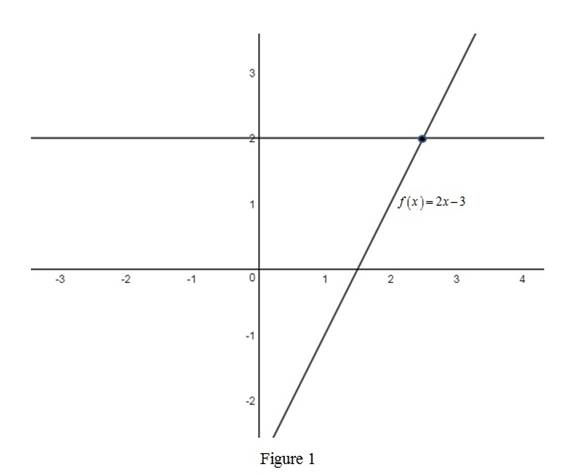# Whether the statement, if f is continuous on ( a , b ) then f attains an absolute maximum value f ( c ) and an absolute minimum value f ( d ) at some numbers c and d in ( a , b ) is true or false.### Single Variable Calculus: Concepts...

4th Edition
James Stewart
Publisher: Cengage Learning
ISBN: 9781337687805### Single Variable Calculus: Concepts...

4th Edition
James Stewart
Publisher: Cengage Learning
ISBN: 9781337687805

#### Solutions

Chapter 4, Problem 3RQ
To determine

## Whether the statement, if f is continuous on (a,b) then f attains an absolute maximum value f(c) and an absolute minimum value f(d) at some numbers c and d in (a,b) is true or false.

Expert Solution

### Explanation of Solution

The given statement is false.

Reason:

Case 1:

If the graph of the function is a line parallel to x-axis, then there will be no absolute minimum or an absolute maximum.

Case 2:

If the graph of the function is a line which is defined on any open interval (a, b), then there will be no absolute minimum or an absolute maximum.From Figure 1, it can be identified that either the horizontal line or a straight line does not attain its absolute minimum or absolute maximum.

Therefore, the given statement is false.

### Have a homework question?

Subscribe to bartleby learn! Ask subject matter experts 30 homework questions each month. Plus, you’ll have access to millions of step-by-step textbook answers!